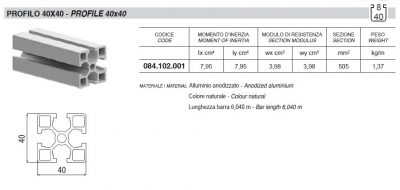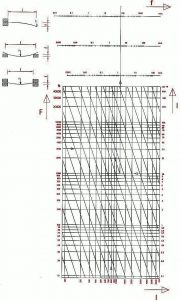## Determining the deflection of a profile under load##Using this diagram we can work out how much a given profile is expected to deflect under a given load condition. The example shown here is for an 800mm long section of profile with a moment of inertia of 10cm^4 and an applied load of 3000N.

Please note that the moment of inertia, Ix & Iy, of each profile is included in the Alusic catalogue along with other information such as section modulus and mass per metre.

Starting along the bottom of the chart, find the length of the profile, in this example the blue vertical line at 800mm. On the left side of the chart find the applied force, in this example the blue horizontal line at 3000N.

Now, from the intersection of the horizontal and vertical lines, draw an oblique parallel line. Then, on the right side of the chart find the moment of inertia of the profile, in this example the blue horizontal line at 10cm^4. Where this horizontal line intersects with the drawn oblique line, draw a vertical line up through the deflection bars above the chart.

The expected maximum deflection of the length of profile can now be read directly from the deflection bar for each of the three figured load cases; cantilever, pinned supports, fixed supports. In this example the expected deflection would be 73mm, 4.7mm, or 1.4mm respectively.

### The Load-Deflection diagram we are using here can be found in the Alusic catalogue. The catalogue is available as a FREE download from our web site. https://www.gapengineering.com.au/ Please be aware that this is an 88MB, 796 page PDF file

Obligatory caveat.

This example provides only a general indication. The exact dimensions of, and loads on, each structure must be calculated by the customer separately from this example.